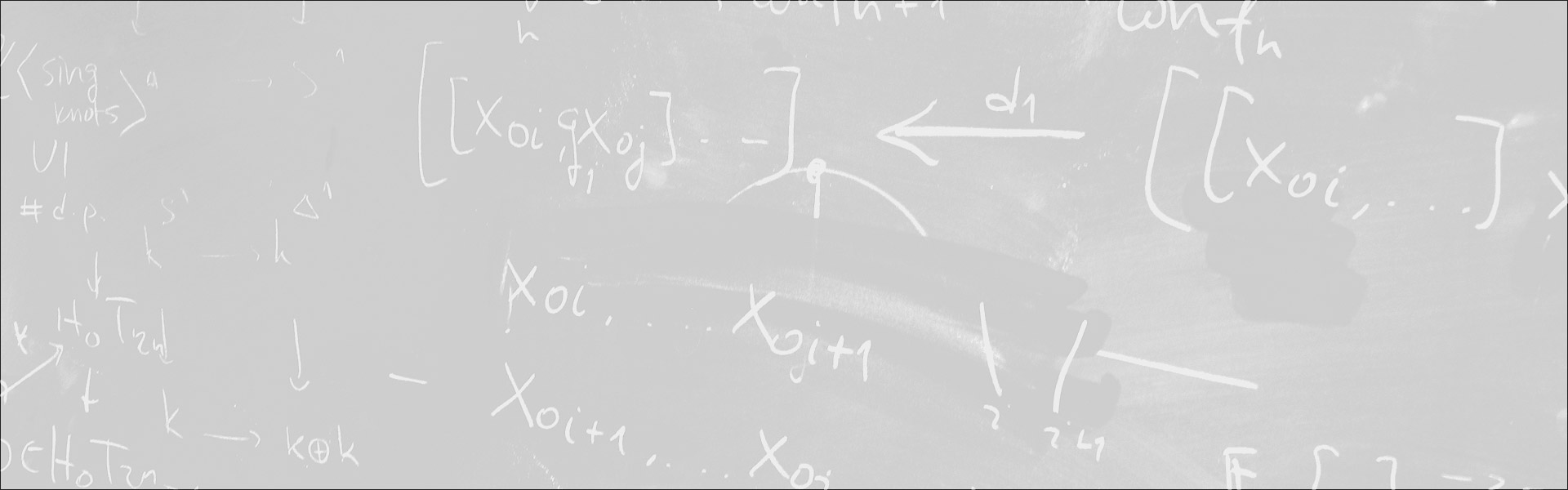Designed and built with care, filled with creative elements

Top# Integer points on analytic sets

/  Évènements• Cet évènement est passé

## Integer points on analytic sets

In 2004 I proved that that if C is a transcendental curve definable in the structure R_{an}, then the number of points on C with integer coordinates of modulus less than H, is bounded by k loglog H for some constsnt k depending only on C. (The situation is vastly different for rational points.) The proof used the fact that such sets C are, in fact, semi-analytic everywhere-including infinity-and so the crux of the matter was to bound the number of solutions to equations of the form

(*)    F(1/n) = 1/mfor n, m integers bounded in modulus by (large) H, and where F is a non-algebraic, analytic function defined on an open interval containing 0.
It turns out that there is probably no generalization of the 2004 result for arbitrary R_{an}-definable sets (which need not be globally, or even locally, semi-analytic) but inspired by observations of Gareth Jones and Gal Binyamini, the three of us began looking at equations of the form (*) in many variables and I shall be reporting on our results.

- Séminaire Géométrie et théorie des modèles

#### Détails :

Orateur / Oratrice : Alex Wilkie
Date : 9 décembre 2022
Horaire : 16h00 - 17h30
Lieu : Salle W (Toits du DMA)